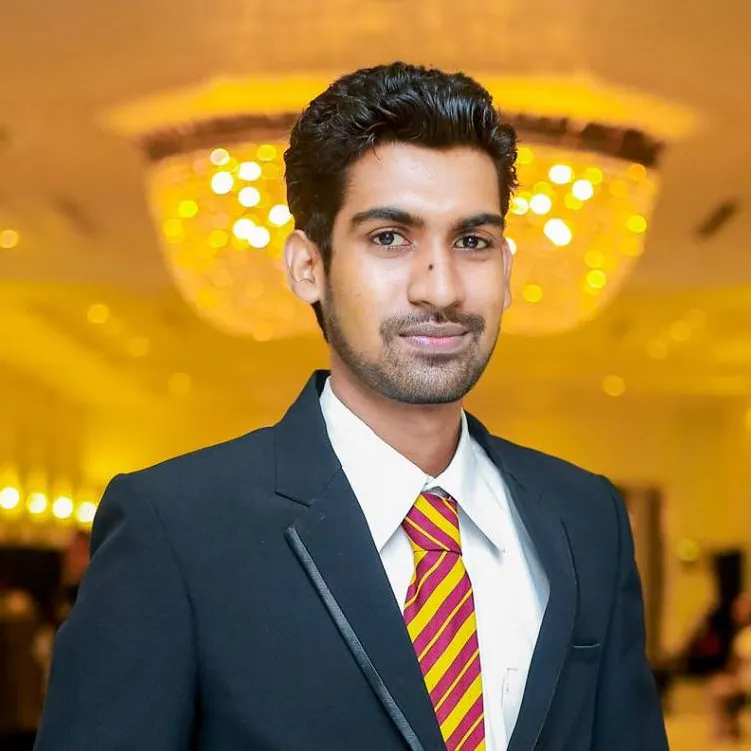Portable Environment Monitor

A handheld monitor for young scientists to get familiar with various aspects of the surrounding environment.

IntermediateFull instructions provided5 hours3,164

Things used in this project

Hardware components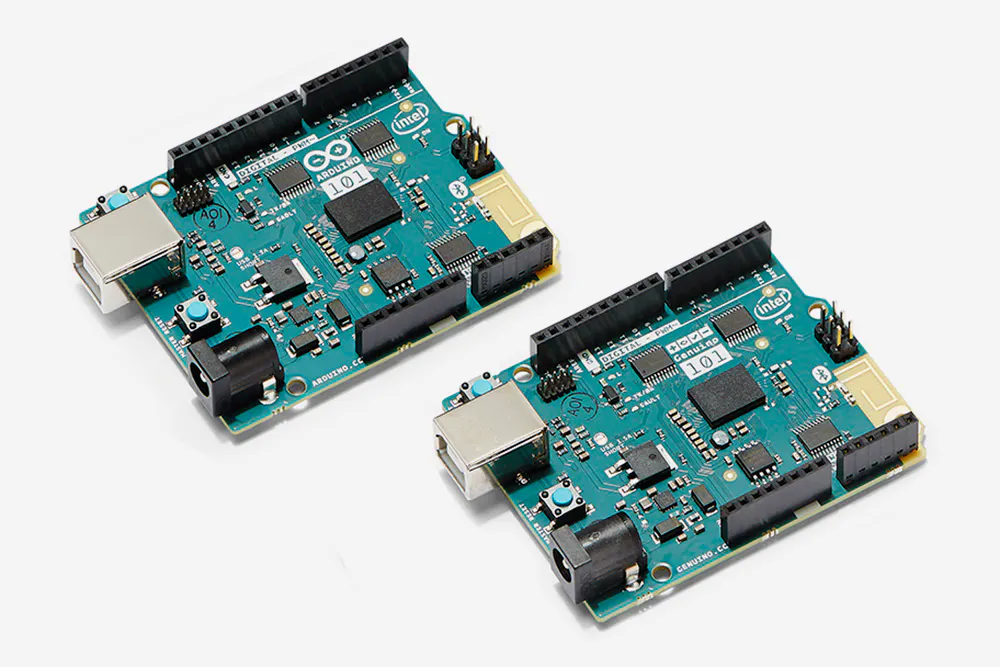Arduino 101 & Genuino 101
×1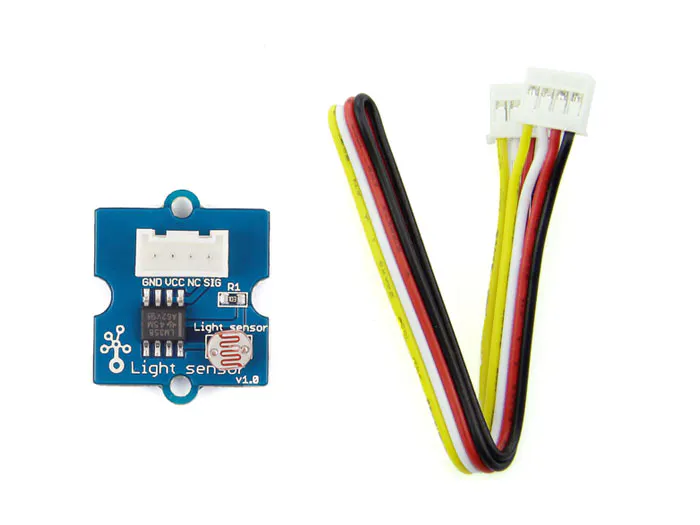Seeed Grove - Light Sensor
×1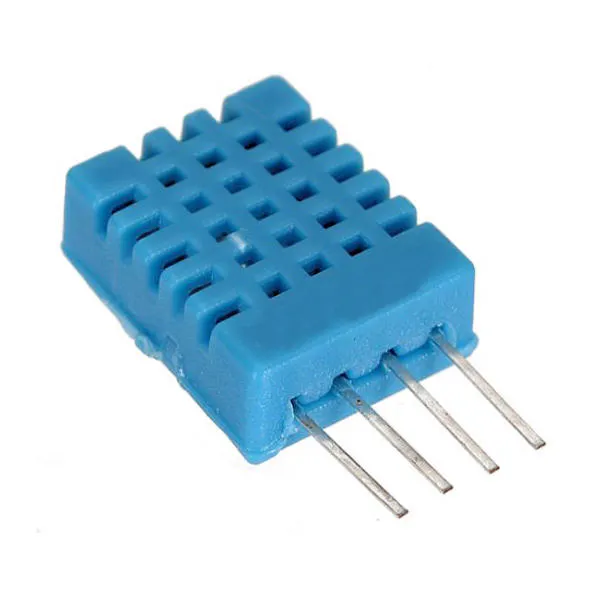DHT11 Temperature & Humidity Sensor (4 pins)
×1
 Sparkfun Sound Detector
×1
 Seeed Grove LCD
×1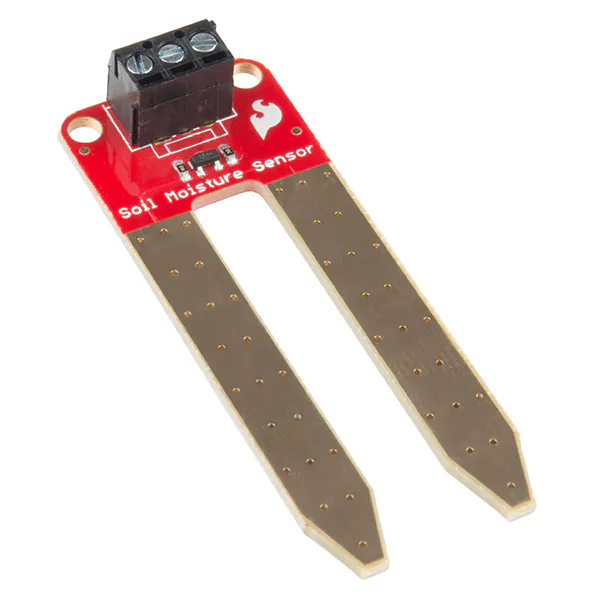SparkFun Soil Moisture Sensor (with Screw Terminals)
×1
 Seeed Grove Button
×1
 Seeed Grove universal cables
×1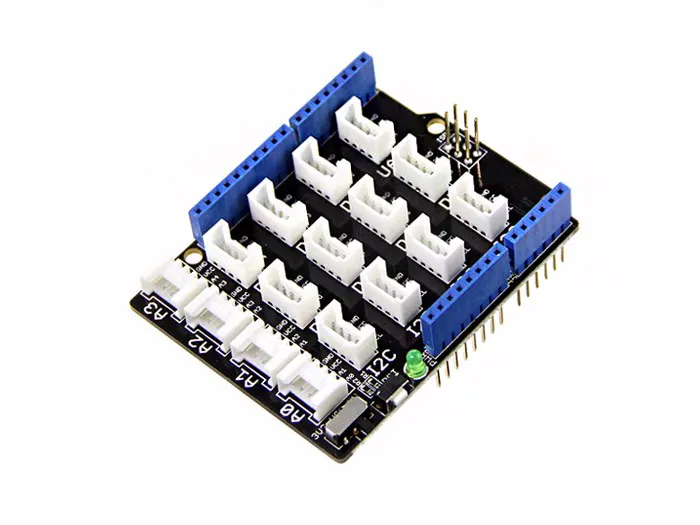Seeed Base Shield V2
×1

Software apps and online servicesArduino IDE

Code

Basic_1.ino

Arduino
#include "DHT.h"         //include DHT library
#define DHTPIN 3         //define as DHTPIN the Pin 3 used to connect the Sensor
#define DHTTYPE DHT11    //define the sensor used(DHT11)
#define light A0
#define noise A1
#define moist A2
#define button 8
DHT dht(DHTPIN, DHTTYPE);//create an instance of DHT
#include <Wire.h>
#include "rgb_lcd.h"
#include "math.h"

rgb_lcd lcd;

const int colorR = 0;
const int colorG = 30;
const int colorB = 55;

int page = 0;

void setup()
{
// set up the LCD's number of columns and rows:
Serial.begin(9600);
lcd.begin(16, 2);
dht.begin();
lcd.setRGB(colorR, colorG, colorB);
pinMode(light,INPUT);
pinMode(noise,INPUT);
pinMode(button,INPUT);
pinMode (2,OUTPUT);
pinMode (A3,OUTPUT);
digitalWrite(2,HIGH);
digitalWrite(A3,OUTPUT);
pinMode (1,OUTPUT);
}

void loop()
{
while (page == 0){
first_page();
if (digitalRead(button) == 1)
page++;
}
delay(500);
while (page == 1){
second_page();
if (digitalRead(button) == 1)
page++;
}
delay(500);

while (page == 2){
third_page();
if (digitalRead(button) == 1)
page = 0;
}

delay(500);

}

void first_page(){

int h = dht.readHumidity();    // reading Humidity
int t = dht.readTemperature();
int light_l = 0;

for (int x=0; x<50;x++){
light_l = light_l + analogRead(light);
delay(2);
}

light_l = light_l/50;
lcd.setCursor(12, 1);
lcd.print("  lx");
lcd.setCursor(0, 0);
lcd.print("Temp ");
lcd.setCursor(0, 1);
lcd.print(t);
lcd.setCursor(2, 1);
lcd.print("_C ");

lcd.setCursor(5, 0);
lcd.print("Humid");
lcd.setCursor(6, 1);
lcd.print(h);
lcd.setCursor(8, 1);
lcd.print("%");

lcd.setCursor(11, 0);
lcd.print("Light");
lcd.setCursor(11, 1);
lcd.print(light_l);

}
void second_page(){

int sound = 0;
for(int x = 0;x<10;x++){
sound = sound + analogRead(noise);
}
sound = sound/10;
double dB = 20*(log10(10*sound)) ;
lcd.setCursor(0, 0);
lcd.print("Noise           ");
lcd.setCursor(0, 1);
lcd.print(dB);
lcd.setCursor(5, 1);
lcd.print("            ");
delay(100);

}

void third_page(){
int moist_s = 0;

for (int y = 0; y<100 ; y++){
moist_s = moist_s + analogRead(moist);
delay(2);
}

moist_s = moist_s/100;
lcd.setCursor(0,0);
lcd.print("Water- ");
lcd.setCursor(0,1);
lcd.print("Soil- ");

lcd.setCursor(6,1);
if(moist_s>900) lcd.print("Dry    ");
else if(moist_s>650) lcd.print("Medium");
else lcd.print("High   ");

lcd.setCursor(7,0);
if(moist_s>750) lcd.print("No      ");
else if(moist_s>600) lcd.print("Distill ");
else if(moist_s>500) lcd.print("Drinking");
else if(moist_s>350) lcd.print("Fish    ");
else lcd.print("Polluted");

}

DHT11.ino

Arduino
// DHT11 Temperature and Humidity Sensors Example
#include "DHT.h"         //include DHT library
#define DHTPIN 3         //define as DHTPIN the Pin 3 used to connect the Sensor
#define DHTTYPE DHT11    //define the sensor used(DHT11)
DHT dht(DHTPIN, DHTTYPE);//create an instance of DHT
/*setup*/
void setup() {
Serial.begin(9600);    //initialize the Serial communication
delay(6000);           //wait 6 seconds
Serial.println("Temperature and Humidity test!");//print on Serial monitor
Serial.println("T(C) \tH(%)");                   //print on Serial monitor
dht.begin();           //initialize the Serial communication
}
/*loop*/
void loop() {

float h = dht.readHumidity();    // reading Humidity
float t = dht.readTemperature(); // read Temperature as Celsius (the default)
// check if any reads failed and exit early (to try again).
if (isnan(h) || isnan(t)) {
Serial.println("Failed to read from DHT sensor!");
return;
}
Serial.print(t, 2);    //print the temperature
Serial.print("\t");
Serial.println(h, 2);  //print the humidity
delay(500);           //wait 2 seconds
}

Kalman.h

C/C++

This software may be distributed and modified under the terms of the GNU
General Public License version 2 (GPL2) as published by the Free Software
Foundation and appearing in the file GPL2.TXT included in the packaging of
this file. Please note that GPL2 Section 2[b] requires that all works based
on this software must also be made publicly available under the terms of
the GPL2 ("Copyleft").

Contact information
-------------------

Kristian Lauszus, TKJ Electronics
Web      :  http://www.tkjelectronics.com
e-mail   :  kristianl@tkjelectronics.com
*/

#ifndef _Kalman_h
#define _Kalman_h

class Kalman {
public:
Kalman() {
/* We will set the variables like so, these can also be tuned by the user */
Q_angle = 0.01;//0.001
Q_bias = 0.0001;//0.003
R_measure = 0.11;//0.03

angle = 0; // Reset the angle
bias = 0; // Reset bias

P = 0; // Since we assume that the bias is 0 and we know the starting angle (use setAngle), the error covariance matrix is set like so - see: http://en.wikipedia.org/wiki/Kalman_filter#Example_application.2C_technical
P = 0;
P = 0;
P = 0;
};
// The angle should be in degrees and the rate should be in degrees per second and the delta time in seconds
double getAngle(double newAngle, double newRate, double dt) {
// KasBot V2  -  Kalman filter module - http://www.x-firm.com/?page_id=145
// Modified by Kristian Lauszus
// See my blog post for more information: http://blog.tkjelectronics.dk/2012/09/a-practical-approach-to-kalman-filter-and-how-to-implement-it

// Discrete Kalman filter time update equations - Time Update ("Predict")
// Update xhat - Project the state ahead
/* Step 1 */
rate = newRate - bias;
angle += dt * rate;

// Update estimation error covariance - Project the error covariance ahead
/* Step 2 */
P += dt * (dt*P - P - P + Q_angle);
P -= dt * P;
P -= dt * P;
P += Q_bias * dt;

// Discrete Kalman filter measurement update equations - Measurement Update ("Correct")
// Calculate Kalman gain - Compute the Kalman gain
/* Step 4 */
S = P + R_measure;
/* Step 5 */
K = P / S;
K = P / S;

// Calculate angle and bias - Update estimate with measurement zk (newAngle)
/* Step 3 */
y = newAngle - angle;
/* Step 6 */
angle += K * y;
bias += K * y;

// Calculate estimation error covariance - Update the error covariance
/* Step 7 */
P -= K * P;
P -= K * P;
P -= K * P;
P -= K * P;

return angle;
};
void setAngle(double newAngle) { angle = newAngle; }; // Used to set angle, this should be set as the starting angle
double getRate() { return rate; }; // Return the unbiased rate

/* These are used to tune the Kalman filter */
void setQangle(double newQ_angle) { Q_angle = newQ_angle; };
void setQbias(double newQ_bias) { Q_bias = newQ_bias; };
void setRmeasure(double newR_measure) { R_measure = newR_measure; };

double getQangle() { return Q_angle; };
double getQbias() { return Q_bias; };
double getRmeasure() { return R_measure; };

private:
/* Kalman filter variables */
double Q_angle; // Process noise variance for the accelerometer
double Q_bias; // Process noise variance for the gyro bias
double R_measure; // Measurement noise variance - this is actually the variance of the measurement noise

double angle; // The angle calculated by the Kalman filter - part of the 2x1 state vector
double bias; // The gyro bias calculated by the Kalman filter - part of the 2x1 state vector
double rate; // Unbiased rate calculated from the rate and the calculated bias - you have to call getAngle to update the rate

double P; // Error covariance matrix - This is a 2x2 matrix
double K; // Kalman gain - This is a 2x1 vector
double y; // Angle difference
double S; // Estimate error
};

#endif

Final Code

Arduino
#include "DHT.h"         //include DHT library
#define DHTPIN 3         //define as DHTPIN the Pin 3 used to connect the Sensor
#define DHTTYPE DHT11    //define the sensor used(DHT11)
#define light A0
#define noise A1
#define moist A2
#define button 8
DHT dht(DHTPIN, DHTTYPE);//create an instance of DHT
#include <Wire.h>
#include "rgb_lcd.h"
#include "math.h"
#include <CurieIMU.h>
#include "Kalman.h"

Kalman kalmanX; // Create the Kalman instances
Kalman kalmanY;

float accX, accY, accZ;
float gyroX, gyroY, gyroZ;

double gyroXangle, gyroYangle;
double compAngleX, compAngleY;
double kalAngleX, kalAngleY;

rgb_lcd lcd;
uint32_t timer;

const int colorR = 0;
const int colorG = 30;
const int colorB = 55;

int page = 0;

void setup()
{
// set up the LCD's number of columns and rows:
Serial.begin(9600);
lcd.begin(16, 2);
dht.begin();
lcd.setRGB(colorR, colorG, colorB);
pinMode(light,INPUT);
pinMode(noise,INPUT);
pinMode(button,INPUT);
pinMode (2,OUTPUT);
pinMode (A3,OUTPUT);
digitalWrite(2,HIGH);
digitalWrite(A3,OUTPUT);
pinMode (1,OUTPUT);
CurieIMU.begin();
CurieIMU.setAccelerometerRange(2);
CurieIMU.setGyroRange(250);
delay(100); // Wait for sensor to stabilize

double roll  = atan2(accY,accZ) * RAD_TO_DEG;
double pitch = atan(-accX / sqrt(accY * accY + accZ * accZ)) * RAD_TO_DEG;
//double yaw = atan (accZ/sqrt(accX*accX + accZ*accZ)) * RAD_TO_DEG;
kalmanX.setAngle(roll); // Set starting angle
kalmanY.setAngle(pitch);
gyroXangle = roll;
gyroYangle = pitch;
}

void loop()
{
while (page == 0){
first_page();
if (digitalRead(button) == 1)
page++;
}
delay(500);
while (page == 1){
second_page();
if (digitalRead(button) == 1)
page++;
}
delay(500);

while (page == 2){
third_page();
if (digitalRead(button) == 1)
page = 0;
}

delay(500);

}

void first_page(){

int h = dht.readHumidity();    // reading Humidity
int t = dht.readTemperature();
int light_l = 0;

for (int x=0; x<50;x++){
light_l = light_l + analogRead(light);
delay(2);
}

light_l = light_l/50;
lcd.setCursor(12, 1);
lcd.print("  lx");
lcd.setCursor(0, 0);
lcd.print("Temp ");
lcd.setCursor(0, 1);
lcd.print(t);
lcd.setCursor(2, 1);
lcd.print("_C ");

lcd.setCursor(5, 0);
lcd.print("Humid");
lcd.setCursor(6, 1);
lcd.print(h);
lcd.setCursor(8, 1);
lcd.print("%");

lcd.setCursor(11, 0);
lcd.print("Light");
lcd.setCursor(11, 1);
lcd.print(light_l);

}
void second_page(){

int sound = 0;
for(int x = 0;x<10;x++){
sound = sound + analogRead(noise);
}
sound = sound/10;
double dB = 20*(log10(10*sound)) ;
lcd.setCursor(0, 0);
lcd.print("Noise ");
lcd.setCursor(0, 1);
lcd.print(dB,0);
lcd.setCursor(2, 1);
lcd.print("dB");
lcd.setCursor(6, 0);
lcd.print("Roll ");
lcd.setCursor(11, 0);
lcd.print("Pitch");

for (int z=0; z<10;z++){

double dt = (double)(micros() - timer) / 1000000; // Calculate delta time
timer = micros();

double roll  = atan2(accY,accZ) * RAD_TO_DEG;
double pitch = atan(-accX / sqrt(accY * accY + accZ * accZ)) * RAD_TO_DEG;
//double yaw = atan2(accZ,sqrt(accX*accX + accZ*accZ)) * RAD_TO_DEG;

double gyroXrate = gyroX / 131.0; // Convert to deg/s
double gyroYrate = gyroY / 131.0; // Convert to deg/s

if ((roll < -90 && kalAngleX > 90) || (roll > 90 && kalAngleX < -90)) {
kalmanX.setAngle(roll);
compAngleX = roll;
kalAngleX = roll;
gyroXangle = roll;
} else
kalAngleX = kalmanX.getAngle(roll, gyroXrate, dt); // Calculate the angle using a Kalman filter

if (abs(kalAngleX) > 90)
gyroYrate = -gyroYrate; // Invert rate, so it fits the restriced accelerometer reading
kalAngleY = kalmanY.getAngle(pitch, gyroYrate, dt);

gyroXangle += gyroXrate * dt; // Calculate gyro angle without any filter
gyroYangle += gyroYrate * dt;
//gyroXangle += kalmanX.getRate() * dt; // Calculate gyro angle using the unbiased rate
//gyroYangle += kalmanY.getRate() * dt;

compAngleX = 0.93 * (compAngleX + gyroXrate * dt) + 0.07 * roll; // Calculate the angle using a Complimentary filter
compAngleY = 0.93 * (compAngleY + gyroYrate * dt) + 0.07 * pitch;

if (gyroXangle < -180 || gyroXangle > 180)
gyroXangle = kalAngleX;
if (gyroYangle < -180 || gyroYangle > 180)
gyroYangle = kalAngleY;

delay(10);
}

lcd.setCursor(10,1);
lcd.print("  ");
lcd.setCursor(6,1);
lcd.print(kalAngleX,1);
lcd.setCursor(15,1);
lcd.print(" ");
lcd.setCursor(12,1);
lcd.print(kalAngleY,1);

}

void third_page(){
int moist_s = 0;

for (int y = 0; y<100 ; y++){
moist_s = moist_s + analogRead(moist);
delay(2);
}

moist_s = moist_s/100;
lcd.setCursor(0,0);
lcd.print("Water- ");
lcd.setCursor(0,1);
lcd.print("Soil- ");

lcd.setCursor(6,1);
if(moist_s>900) lcd.print("Dry       ");
else if(moist_s>650) lcd.print("Medium");
else lcd.print("High   ");

lcd.setCursor(7,0);
if(moist_s>750) lcd.print("No       ");
else if(moist_s>600) lcd.print("Distill ");
else if(moist_s>500) lcd.print("Drinking");
else if(moist_s>350) lcd.print("Fish    ");
else lcd.print("Polluted");

}

Credits

Chathuranga Liyanage

1 project • 20 followers
Undergraduate in Electronic and Telecommunication Engineering